# Algebra 1 : How to factor the quadratic equation

## Example Questions

1 3 Next →

### Example Question #231 : Equations / Inequalities

Solve the following equation by factoring.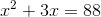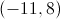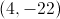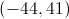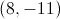Explanation:Begin by setting the equation equal to 0 by subtracting 88 from both sides.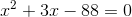Now that the equation is in the form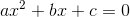, find two integers that sum toand have a product of.

For this equation, those integers areand.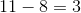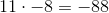Therefore, the solutions to this equation are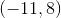### Example Question #22 : How To Factor The Quadratic Equation

Solve the following equation by factoring.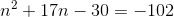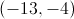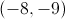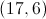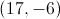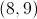Explanation:Begin by setting the equation equal to zero by adding 105 to each side.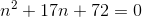For an equation in the form, where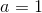, find two integers that have a sum ofand a product of.

For this equation, that would be 8 and 9.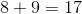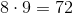Therefore, the solutions to this equation are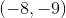1 3 Next →